×#### Thank you for registering.

One of our academic counsellors will contact you within 1 working day.

Click to Chat

1800-1023-196

+91-120-4616500

CART 0

• 0

MY CART (5)

Use Coupon: CART20 and get 20% off on all online Study Material

ITEM
DETAILS
MRP
DISCOUNT
FINAL PRICE
Total Price: Rs.

There are no items in this cart.
Continue Shopping• Complete JEE Main/Advanced Course and Test Series
• OFFERED PRICE: Rs. 15,900
• View Details

```Revision Notes on Rational Numbers

Rational Numbers

A number is called Rational if it can be expressed in the form p/q where p and q are integers (q > 0). It includes all natural, whole number and integers.

Example: 1/2, 4/3, 5/7,1 etc.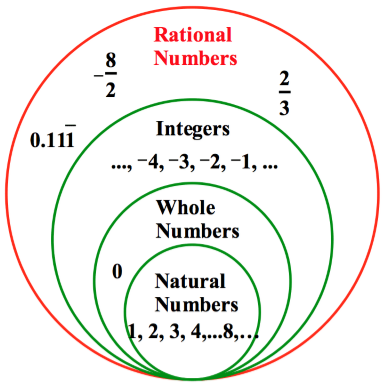Natural Numbers

All the positive integers from 1, 2, 3,……, ∞.

Whole Numbers

All the natural numbers including zero are called Whole Numbers.

Integers

All negative and positive numbers including zero are called Integers.

Properties of Rational Numbers

1. Closure Property

This shows that the operation of any two same types of numbers is also the same type or not.

a. Whole Numbers

If p and q are two whole numbers then

Operation
Subtraction
Multiplication
Division

Whole number

p + q will also be the whole number.

p – q will not always be a whole number.

pq will also be the whole number.

p ÷ q will not always be a whole number.

Example

6 + 0 = 6

8 – 10 = – 2

3 × 5 = 15

3 ÷ 5 = 3/5

Closed or Not

Closed

Not closed

Closed

Not closed

b. Integers

If p and q are two integers then

Operation
Subtraction
Multiplication
Division

Integers

p+q will also be an integer.

p-q will also be an integer.

pq will also be an integer.

p ÷ q will not always be an integer.

Example

- 3 + 2 = – 1

5 – 7 = – 2

- 5 × 8 = – 40

- 5 ÷ 7  = – 5/7

Closed or not

Closed

Closed

Closed

Not  closed

c. Rational Numbers

If p and q are two rational numbers then

Operation
Subtraction
Multiplication
Division

Rational Numbers

p + q will also be a rational number.

p – q will also be a rational number.

pq will also be a rational number.

p ÷ q will not always be a rational number

Example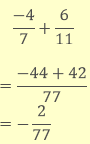p ÷ 0

= not defined

Closed or Not

Closed

Closed

Closed

Not closed

2. Commutative Property

This shows that the position of numbers does not matter i.e. if you swap the positions of the numbers then also the result will be the same.

a. Whole Numbers

If p and q are two whole numbers then

Operation
Subtraction
Multiplication
Division

Whole number

p + q = q + p

p – q ≠ q – p

p × q = q × p

p ÷ q ≠ q ÷ p

Example

3 + 2 = 2 + 3

8 –10 ≠ 10 – 8 – 2 ≠ 2

3 × 5 = 5 × 3

3 ÷ 5 ≠ 5 ÷ 3

Commutative

yes

No

yes

No

b. Integers

If p and q are two integers then

Operation
Subtraction
Multiplication
Division

Integers

p + q = q + p

p – q ≠ q – p

p × q = q × p

p ÷ q ≠ q ÷ p

Example

True

5 – 7 = – 7 – (5)

- 5 × 8 = 8 × (–5)

- 5 ÷ 7 ≠ 7 ÷ (-5)

Commutative

yes

No

yes

No

c. Rational Numbers

If p and q are two rational numbers then

Operation
Subtraction
Multiplication
Division

Rational numbers

p + q = q + p

p –q ≠ q - p

p × q = q × p

p ÷ q ≠ q ÷ p

Example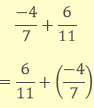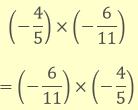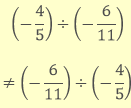Commutative

yes

No

yes

No

3. Associative Property

This shows that the grouping of numbers does not matter i.e. we can use operations on any two numbers first and the result will be the same.

a. Whole Numbers

If p, q and r are three whole numbers then

Operation
Subtraction
Multiplication
Division

Whole number

p + (q + r) = (p + q) + r

p – (q – r) = (p – q) – r

p × (q × r) = (p × q) × r

p ÷ (q ÷ r)  ≠ (p ÷ q) ÷ r

Example

3 + (2 + 5) = (3 + 2) + 5

8 – (10 – 2) ≠ (8 -10) – 2

3 × (5 × 2) = (3 × 5) × 2

10 ÷ (5 ÷ 1) ≠ (10 ÷ 5) ÷ 1

Associative

yes

No

yes

No

b. Integers

If p, q and r are three integers then

Operation
Integers
Example
Associative

p + (q + r) = (p + q) + r

(– 6) + [(– 4)+(–5)] = [(– 6) +(– 4)] + (–5)

Yes

Subtraction

p – (q – r) = (p – q) – r

5 – (7 – 3) ≠ (5 – 7) – 3

No

Multiplication

p × (q × r) = (p × q) × r

(– 4) × [(– 8) ×(–5)] = [(– 4) × (– 8)] × (–5)

Yes

Division

p ÷ (q ÷ r) ≠ (p ÷ q) ÷ r

[(–10) ÷ 2] ÷ (–5) ≠ (–10) ÷ [2 ÷ (– 5)]

No

c. Rational Numbers

If p, q and r are three rational numbers then

Operation
Integers
Example
Associative

p + (q + r) = (p + q) + r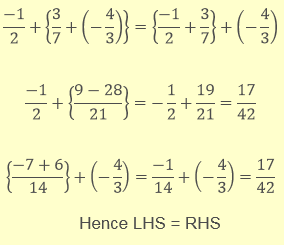yes

Subtraction

p – (q – r) = (p – q) – r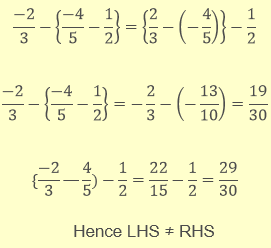No

Multiplication

p × (q × r) = (p × q) × r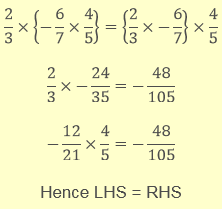yes

Division

p ÷ (q ÷ r)  ≠ (p ÷ q) ÷ r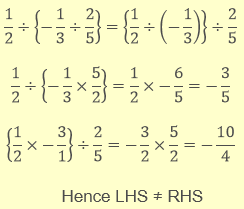No

The Role of Zero in Numbers (Additive Identity)

Zero is the additive identity for whole numbers, integers and rational numbers.

Identity

Example

Whole number

a + 0 = 0 + a = a

Addition of zero to whole number

2 + 0 = 0 + 2 = 2

Integer

b + 0 = 0 + b = b

Addition of zero to an integer

False

Rational number

c + 0 = 0 + c = c

Addition of zero to a rational number

2/5 + 0 = 0 + 2/5 = 2/5

The Role of one in Numbers (Multiplicative Identity)

One is the multiplicative identity for whole numbers, integers and rational numbers.

Identity

Example

Whole number

a ×1 = a

Multiplication of one to the whole number

5 × 1 = 5

Integer

b × 1= b

Multiplication of one to an integer

- 5 × 1 = – 5

Rational Number

c × 1= c

Multiplication of one to a rational number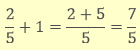Negative of a Number (Additive Inverse)

Identity

Example

Whole number

a +(- a) = 0

Where a is a  whole number

5 + (-5) = 0

Integer

b +(- b) = 0

Where b is an integer

True

Rational number

c + (-c) = 0

Where c is a rational number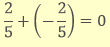Reciprocal (Multiplicative Inverse)

The multiplicative inverse of any rational number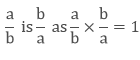Example

The reciprocal of 4/5 is 5/4.

Distributivity of Multiplication over Addition and Subtraction for Rational Numbers

This shows that for all rational numbers p, q and r

1. p(q + r) = pq + pr

2. p(q – r) = pq – pr

Example

Check the distributive property of the three rational numbers 4/7,-( 2)/3 and 1/2.

Solution

Let’s find the value ofThis shows that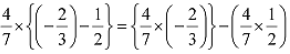Representation of Rational Numbers on the Number Line

On the number line, we can represent the Natural numbers, whole numbers and integers as followsRational Numbers can be represented as followsRational Numbers between Two Rational Numbers

There could be n number of rational numbers between two rational numbers. There are two methods to find rational numbers between two rational numbers.

Method 1

We have to find the equivalent fraction of the given rational numbers and write the rational numbers which come in between these numbers. These numbers are the required rational numbers.

Example

Find the rational number between 1/10 and 2/10.

Solution

As we can see that there are no visible rational numbers between these two numbers. So we need to write the equivalent fraction.

2/10 = 20/100((multiply the numerator and denominator by 10)

Hence, 2/100, 3/100, 4/100……19/100 are all the rational numbers between 1/10 and 2/10.

Method 2

We have to find the mean (average) of the two given rational numbers and the mean is the required rational number.

Example

Find the rational number between 1/10 and 2/10.

Solution

To find mean we have to divide the sum of two rational numbers by 2.3/20 is the required rational numbers and we can find more by continuing the same process with the old and the new rational number.

Remark: 1. This shows that if p and q are two rational numbers then (p + q)/2 is a rational number between p and q so that

p < (p + q)/2 < q.

2. There are infinite rational numbers between any two rational numbers.
```### Course Features

• 728 Video Lectures
• Revision Notes
• Previous Year Papers
• Mind Map
• Study Planner
• NCERT Solutions
• Discussion Forum
• Test paper with Video Solution